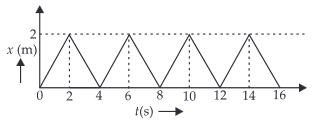#### The figure shows the position - timegraph of one-dimensional motion of a body of mass 0.4 kg. The magnitude of each impulse isOption 1) 0.2 N s Option 2) 0.4 N s Option 3) 0.8 N s Option 4) 1.6 N sAs we learnt in

Impulse Momentum Theorem -- wherein

Ifis increased, average force is decreased

Initial velocity,Final velocity,Initial MomentumFinal MomentumImpulse = Change in Momentum =Correct option is .3

Option 1)

0.2 N s

This is an incorrect option.

Option 2)

0.4 N s

This is an incorrect option.

Option 3)

0.8 N s

This is the correct option.

Option 4)

1.6 N s

This is an incorrect option.

## Crack JEE Main with "AI Coach"

• HD Video Lectures
• Unlimited Mock Tests
• Faculty Support1.6Ns